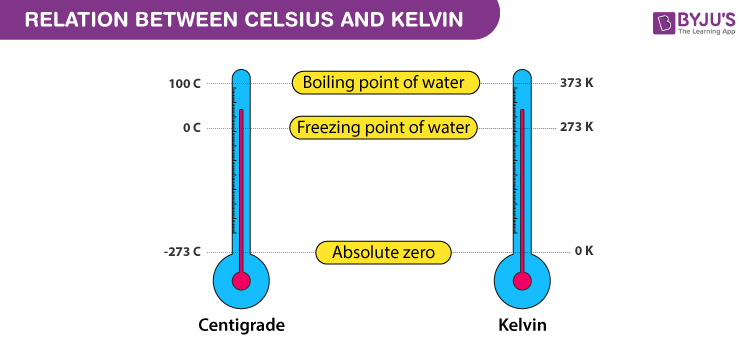# Kelvin Scale

## What is Kelvin Scale?

The absolute, or kelvin, scale Temperatures exist which are much colder than the freezing point of ice, 0°C on the Celsius scale.

Kelvin wisely proposed that at first the temperature interval from the ice point to the steam point be defined as 100 degrees, consistent with earlier centigrade scales. All thermometers have a scale on them which we read to find temperature, called a temperature scale.

Kelvin (K) Ordinarily, we measure temperature in degree centigrade (°C). In this scale, the two fixed temperatures taken are ice-point 0°C and the steam point (100°C) and the interval between these two is divided into 100 equal parts.

## Recommended Videos## History of Kelvin Scale

In 1848, Lord Kelvin defined an absolute temperature scale based on the Carnot cycle which was later named after him as Kelvin’s absolute temperature scale. In Kelvin’s scale, the zero point is 273.15 below that of the Celsius scale. The true origin of the universe, if it had one, remains a mystery for the present and likely will remain one far into the future.Each division in the kelvin scale called a kelvin (K) is equal to a degree on the Celsius scale, but the difference is where zero is. In the Celsius scale 0o is the freezing point of water while in the Kelvin scale the zero point is at absolute zero. Therefore, 0oK is equal to -273.15oC, 0oC is equal to 273.15 kelvins. The Kelvin scale is used for very low or very high temperatures when water is not involved.

The kelvin scale of heat measurement differs from the centigrade and Fahrenheit scales in that it starts from absolute zero, the lowest possible temperature. In which there is no energy whatsoever present. The centigrade scale assigns 0o to the temperature at which water freezes and 100o to the temperature at which it boils, while in the Fahrenheit scale water freezes at 32o and boils at 212o. The Kelvin scale otherwise follows the centigrade scale. Absolute zero is -273.15oC and -459.67oF, water freezes at 273.15oK and boils at 373.15oK

## Kelvin Scale Formula

A mathematical equation that gives the relationship between the Celsius and Kelvin scales:

K = °C + 273

This simple formula states that to find Kelvin temperature (K).

This scale is also called SI scale of temperature. It is mostly used by the scientific community. It was designed to honour Lord Kelvin, a renowned physicist. The lower standard point on this scale is 273K and upper standard point is 373K. There 273 divisions below lower standard point. Its 0K is equal to -273oC. To convert Celsius temperature to kelvin temperature following formulas is used.

Temperature in Kelvin = Temperature in oC + 273

For example, 30oC = (30+273) = 303K

Conversely, (353-273) = 80oC

To convert temperature from Celsius scale to kelvin scale the value is increased numerically by 273. Therefore, the temperature on the kelvin scale and Celsius scale are related to each other as follows.

T(K) = T(oC) + 273

The Celsius and the kelvin scales are used all over the world for scientific measurements. These scales are also found to be more convenient than the scales introduced by Fahrenheit and Reaumer which were commonly used till recently.

The following formula is used for the conversion between different temperature scales.

K-273/100 = C/100 = F-32/180 = R/80

## Solved Problems

1. Convert 25oC into Kelvin.

Solution:

Temperature in K = Temperature in oC + 273

= 25 + 273

= 293 K

2. Convert 104oF into Kelvin.

Solution:

K-273/100 = F-32/180
K= (F-32)5/9 +273
= (104-32)5/9 +273
= 72 x 5/9 + 273
=40+273 = 313K

3. A temperature x is recorded both on Celsius and Fahrenheit scale. What is the value of x?

Solution:

We know

C/5 = F-32/9

= x/5 = x-32/9

= 9x = 5x – 160

4x = -160

Therefore, x = -40

## Frequently Asked Questions on Kelvin Scale

### What is the Kelvin scale used for?

Kelvin is also used in colour temperature determination and is usually used in lighting. In an application for lighting, Kelvin temperature reflects the colour temperature related to an object’s physical temperature, such as white, blue or bright red.

### Is Negative Kelvin possible?

The particles cease travelling at zero kelvin minus 273 degrees centigrade and all disturbance disappears. So nothing on the Kelvin scale can be colder than absolute zero. In the laboratory, physicists have now produced an atomic gas that has negative Kelvin values though.

### What is the unit of Kelvin?

The kelvin, unit of thermodynamic temperature, is the fraction 1/273.16 of the triple point of water’s thermodynamic temperature. The kelvin and the degree Celsius are both the units of the 1990 International Temperature Scale, introduced in 1989 by the CIPM.

### Why do we use Kelvin instead of Celsius?

Kelvin 0 degrees stands for zero kinetic energy or temperature. Changes in Celsius or Fahrenheit are not directly related to kinetic energy or volume because these scales do not begin at zero. Scientists use the Kelvin scale because it is an absolute measure of temperature and is directly related to kinetic energy and volume.

### What is the difference between Kelvin and Celsius?

The same magnitude for degrees Celsius (° C) and kelvins (K). The only difference between the scales is their point of departure: 0 K is “absolute zero,” while 0°C is the point of freezing. Through adding 273.15 one can convert degrees Celsius to kelvins; so the boiling point of water, 100°C, is 373.15 K.

Test your Knowledge on Kelvin temperature scale!

#### 1 Comment

1. kirankhurshid

i like your explanation of topics because you explain in very easy method.